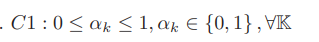# How to express this formula in cvxThis formula is one of the constraints, and I don’t know how to express it in cvx

If `alpha` is a vector variable or expression to be constrained to the continuous interval `[0,1]`, use `0 <= alpha <= 1`.

However, if `{0,1} ` actually means that alpha can only takes the value 0 or 1, and not any value in the continuous interval `[0,1]`, you should declare `alpha` as binary, in which case specifying `0 <= alpha <= 1` is redundant

It actually means that alpha can only takes the value 0 or 1 (the each element in the matrix only takes the value 0 or 1). How should I declare each element as binary?

If `alpha` is a k by 1 vector variable:

`variable alpha(k) binary`

Thanks a lot!!!